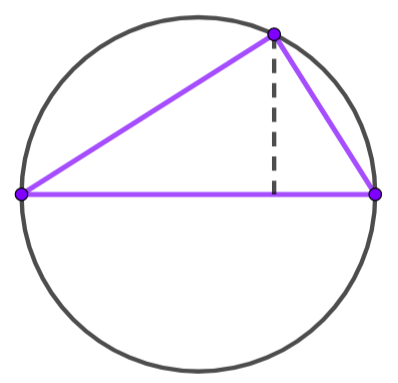# The Theorem

The namesake of this website is…

Angle Inscribed in a Semicircle Theorem:An angle inscribed in a semicircle is a right angle.

Equivalent forms:

• If an angle is inscribed in a semicircle, then it is a right angle.
• If an angle is determined by the endpoints of a diameter and a third point on the circle, as the vertex, then the angle is a right angle.

Converse:

An inscribed angle which is a right angle is inscribed in a semicircle.

Equivalent forms:

• If a right angle is an inscribed angle of a circle, then it is inscribed in a semicircle.
• If a right angle, ∠ABC, is an inscribed angle of a circle, then segment AB is a diameter of the circle.

This is my favorite theorem in mathematics!

It is also named Thale’s Theorem. It is a special case of the inscribed angle theorem.

It is surprising how many times and places it can be used.  One rather obscure, but profound, example is the proof of Circle of Apollonius using the angle bisector theorem.

Jim Olsen

See example at http://www.mathopenref.com/semiinscribed.html